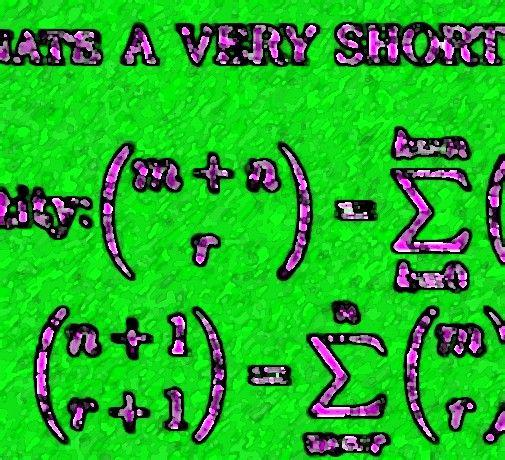## Sunday, 17 January 2010

### READER'S CORNER (I)A BINOMIAL PLAY:

Our contributor Raymond Rogers has sent an alternate proof for:

$latex \displaystyle det{\bigg[ \binom{i+j+k}{i} \bigg]}_{0\leq i,j \leq n} =\binom{n+k+1}{k+1}$

This expression is identical to the one found in the post Binomial Matrix (III), but here the matrices are indexed from zero instead of one.

The proof is entitled A BINOMIAL DETERMINATE,A VERY SHORT PLAY IN THREE ACTS, and it is based on Vandermonde's identity.

Thank you Raymond.

Archives:

Links:
-Raymond Rogers, Lamm´s Equation, Confluent Hypergeometric Equations- Lamm´s Equation Blogspot